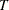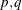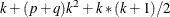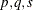# The VARMAX Procedure

### Computational Issues

Subsections:

#### Computational Method

The VARMAX procedure uses numerous linear algebra routines and frequently uses the sweep operator (Goodnight, 1979) and the Cholesky root (Golub and Van Loan, 1983).

In addition, the VARMAX procedure uses the nonlinear optimization (NLO) subsystem to perform nonlinear optimization tasks for the maximum likelihood estimation. The optimization requires intensive computation.

#### Convergence Problems

For some data sets, the computation algorithm can fail to converge. Nonconvergence can result from a number of causes, including flat or ridged likelihood surfaces and ill-conditioned data.

If you experience convergence problems, the following points might be helpful:

• Data that contain extreme values can affect results in PROC VARMAX. Rescaling the data can improve stability.

• Changing the TECH=, MAXITER=, and MAXFUNC= options in the NLOPTIONS statement can improve the stability of the optimization process.

• Specifying a different model that might fit the data more closely and might improve convergence.

#### Memory

Letbe the length of each series, k be the number of dependent variables, p be the order of autoregressive terms, and q be the order of moving-average terms. The number of parameters to estimate for a VARMA() model isAs k increases, the number of parameters to estimate increases very quickly. Furthermore the memory requirement for VARMA() quadratically increases as k andincrease.

For a VARMAX() model and GARCH-type multivariate conditional heteroscedasticity models, the number of parameters to estimate and the memory requirements are considerable.

#### Computing Time

PROC VARMAX is computationally intensive, and execution times can be long. Extensive CPU time is often required to compute the maximum likelihood estimates.